# Affine Alignment of Ultrasound Volumes Using ... - NTNU Open

N/A
N/A
Protected

Share "Affine Alignment of Ultrasound Volumes Using ... - NTNU Open"

Copied!
72
0
0
Vis mer ( sider)

Fulltekst

This is due to the computational requirements of the iterative optimization of 3D image registration algorithms. Quicksilver is a deep learning based system for image registration of MR images of the human brain.

## Image registration

### NCC

Resampling is the part where the moving image is transformed so that it approximates the target image by the transformation found earlier. This is done by calculating the displacement between the moving image and the target image using a third-order B-spline interpolation.

## Geometric spatial transformations

### Spatial transformation of coordinates for 3D images

The resulting transformed image will be a copy of the original image, where the image origin is translated into x voxels in the x-direction, ty voxels in the y-direction, and tzvoxels in the z-direction, as can be seen from equation (2.12c). As can be seen from equation (2.13), shearing is achieved by adding shear factors to the off-diagonal elements of the identity matrix.

### Intensity interpolation

From the figure, it is clear that bilinear interpolation creates a smoother transition between colors, while nearest neighbor interpolation creates a more distorted and pixelated image. An alternative method for Equation (2.16) can be found by assuming that the cube formed by the 8 surrounding corners (as in Figure 2.3) is a unit cube. Using this, the trilinear interpolation method can be simplified to 2 bilinear interpolations and 1 linear interpolation.

Rajon and Bolch (2003) show how this is done by first doing a bilinear interpolation on the x-y plane, followed by a new bilinear interpolation on the x-z plane. This results in 2 points in the y-z plane which can be used to find the intensity value of the desired voxel-through linear interpolation.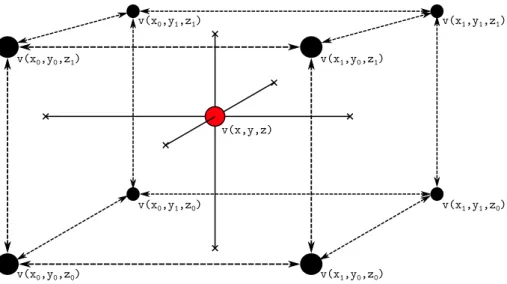Figure 2.2 shows a rotated 2D image which has been interpolated using different inter- inter-polation methods

## Computer vision and deep learning

### Backpropagation

Backpropagation is the name of the algorithm used in deep learning to produce the gradients of the cost function C. The purpose of the backpropagation algorithm is to produce the gradients of the cost function with respect to the wights and biases for each layer (L) in the network. Given a cost function C(a, y) as a function of the output of a neuron,a =f(z), and a certain desired value,y, the gradient with respect to the weights and bias of the layerL can be determined.

Calculating the partial derivatives of the cost function with respect to the weights and biases for each layer (L) in a backward manner (hence the name "backpropagation") leads to the gradient of the cost function,∇C. The gradient is then used to update the weights and biases of the network in a forward pass using an optimization algorithm such as SGD.

## Image registration with deep learning

### Preprocess

It shows that after the histogram equalization, the intensity values ​​of the dark areas of the image were further depressed, while the light areas improved their intensity values. Histogram equalization is done by treating the histogram of the image as the Probability Density Function (PDF)p(Xk), where Xk is a specific intensity level of the image (k = [0,. The transformation that achieves this map is given by: a) in Figure 3.2 corresponds to the histogram of the original image ((a) in Figure 3.1), and (b) in Figure 3.2 corresponds to the histogram of the image after applying histogram equalization ((c) ) in Figure 3.1).

If the sum of the intensity values ​​within the patch is 0, then the entire patch consists only of the black border and the background is removed from consideration. As a result, only the patches containing the part of the ultrasound cone remain.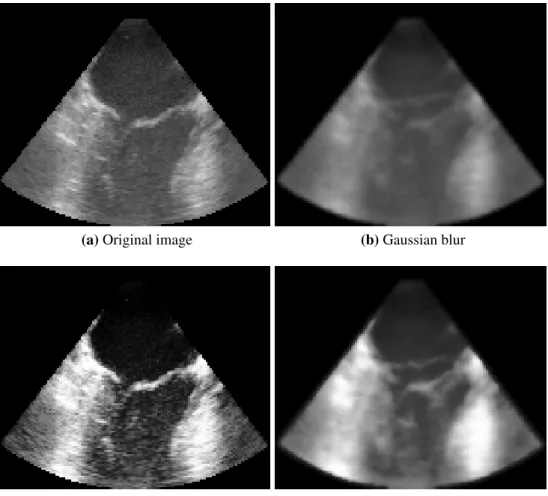Figure 3.1: Comparison of images before and after preprocessing. (a) is the original, unfiltered image.

## Deep learning network

### Full image network

The reasoning behind this is to turn the output of the final CNN into a tensor of shape features×1×1×1. Using the information from Table 3.2, the input of the first fully connected layer becomes 256 (2 times 128). Two pipelines, one for each moving image and one for the target image, consisting of 5 CNN.

The FCNN consists of 3 linear layers where the output of the final layer is the 12 parameters of the affine transformation matrix Equation (2.15). Details from each layer can be seen in Table 3.2 and Table 3.3. due to the focus of the moving image and the CNN target image pipeline).

### Patch based network

The output of the final convolution layer is used as input to the FCNN. Two pipelines, one for each of the moving image and the target image, consisting of 3 CNNs. As for the full image-based model, the output of the two CNN pipelines is used as input to the FCNN, but here without a global pooling layer.

Reshaping this tensor into a 1-dimensional tensor yields an input tensor of size 3456 to the FCNN. Both the full image-based model and the patch-based model are developed in Python using the PyTorch1 library for image registration deep learning implementation.

### Gaussian blur

The Gaussian Blur implementation uses the gaussian_filter function from the SciPy Python library.

Histogram equalization

## Deep learning

### Negative NCC loss function

A specially implemented similarity measure for negative NCC is used in this system as a loss function. The negative of NCC is used to make NCC a minimization problem instead of a maximization problem as stated in equation (2.1). An object of thelibrary.ncc_loss.NCCclass can be initialized at any time, but the calculation of the negative NCC value is performed only when the .forward method is called.

The introduction of bundles means that the calculations of the average and sum values ​​of the images must take bundles into account. By choosing dim=(1,2,3)andkeepdim=True, the system will only consider the average and sum of the images themselves, avoiding cross-batch influence.

## Affine transformation

To see the effect of Gaussian blur, we look at the min, max, mean and variance of the negative NCC between the moving image and the target image. To generate this table, a kernel standard deviation was used, varying between σ ∈ [0.2] in steps of 0.1. Table 5.2 uses the same set of images, but histogram equalization has also been applied.

This is because the purpose of histogram equalization is more directly involved in the deep learning process. Histogram equalization is done to give the images clearer patches within the image that correspond to features that (theoretically) can be easily recognized by a deep learning network.

## Training results

### Full image based network

A set of training images is passed through the network, the backpropagation is calculated from the NCC loss function, and the weights are updated using an optimization algorithm. Note that the patch size parameter is only used in the patch based model and the batch size has been limited to 1 due to limited GPU. A standard deviation of the kernel in the range betweenσ∈[0,2]with0.1increment has been used to blur the images and reduce noise.

### Patch based network

The training of the network was done using a patch with a resolution30×30×30voxels and a batch size of 64. The same set of 71 images was used for the patch-based network as for the full image-based network.

## Test results

As a measure of the system performance, the difference in negative NCC between the moving and target image before and after transformation was used. This gives an indication of the effect that the transformation has on the negative NKK. An example of a transformed image from the test set can be seen in Figure 5.3 and Figure 5.4.

The images in both figures are taken from the same frame (#1) in the test set, but using a different transform prediction method. In Figure 5.3 the transformation is predicted using the full image-based network, while in Figure 5.4 the patch-based network is used.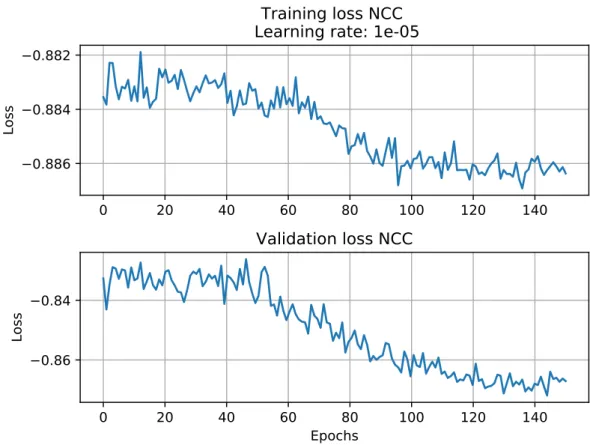Figure 5.2: Training (top) and validation (bottom) loss graphs for training of the patch based network.

## Runtime

No filter was used in (a), only Gaussian blur was used in (b), only histogram equalization was used in (c), and both Gaussian blur and histogram equalization were used in (d). All these loss graphs are generated by patch-based network training. that the histogram equalization allowed the system to recognize the features of the ultrasound images and use them to find affine transformations to train the network. Comparing the initial negative NCC values ​​and the convergent negative NCC values ​​for (c) and (d), we can see that the starting point when only histogram equalization was used is −0.828, while .

The difference basically comes from the effect Gaussian blur has on the negative NCC as shown in Table 5.1 and Table 5.2. The introduction of Gaussian blur in addition to histogram equalization provides an improvement in training loss of 66.7%.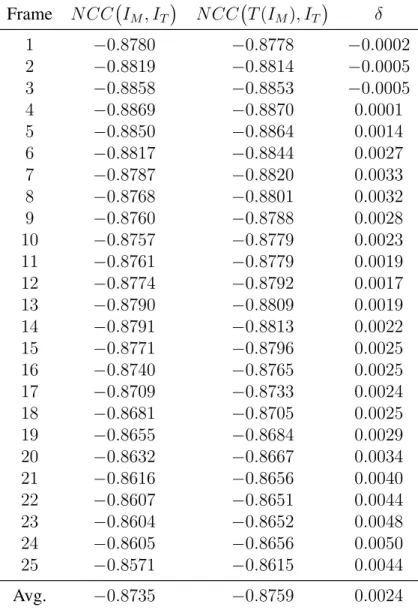Table 5.6: Change in negative NCC before and after transformation as well as the difference of the two for the patch based model.

## Performance

### Overtraining

An overfitted model tends to remember the structures of the training images instead of generalizing the features that represent the training images. From the top graph in this figure, we can see that the training loss decreases throughout the training process and converges towards -0.879 around epoch 120. This is because the network begins to assume that the input will always be the training data, and thus finds a solution that works well for all training images but does not work in the general case.

The training loss (upper part) is decreasing throughout the training process, but the validation loss (lower part) reaches a point where it starts to increase and increases again. This is due to overfitting, where the network remembers the structures of the training images instead of generalizing.

## Runtime analysis

By omitting the Gaussian blur pre-process, the calculation speed of the system will increase by 17.5% and 15.4%, respectively. In a real-time environment, the images would be fed into the system from a live stream and thus loading and storing the images externally for pre-processing purposes would not be necessary. Optimization when it comes to how images are loaded into memory can be done here as well to increase the speed of the calculation.

Since histogram equalization is a necessary preprocessing filter to ensure training convergence, it would be harder to argue for omitting histogram equalization. Both Gaussian blur and histogram equalization are implemented using the Numpy and SciPy libraries, which do not have built-in GPU acceleration.

## Future work

Further training and refinement of the whole image-based model is needed to see the potential of this method. Optimizing Gaussian blur and histogram equalization for GPE-accelerated implementation could significantly reduce computational requirements. This package deals with the calculation of the gradient of the transformed moving image with respect to the target image.

Enabling these options tells PyTorch to calculate the gradient of the output with respect to these tensors. It functionally allows calculation of the gradient of complicated mathematical functions to be done automatically.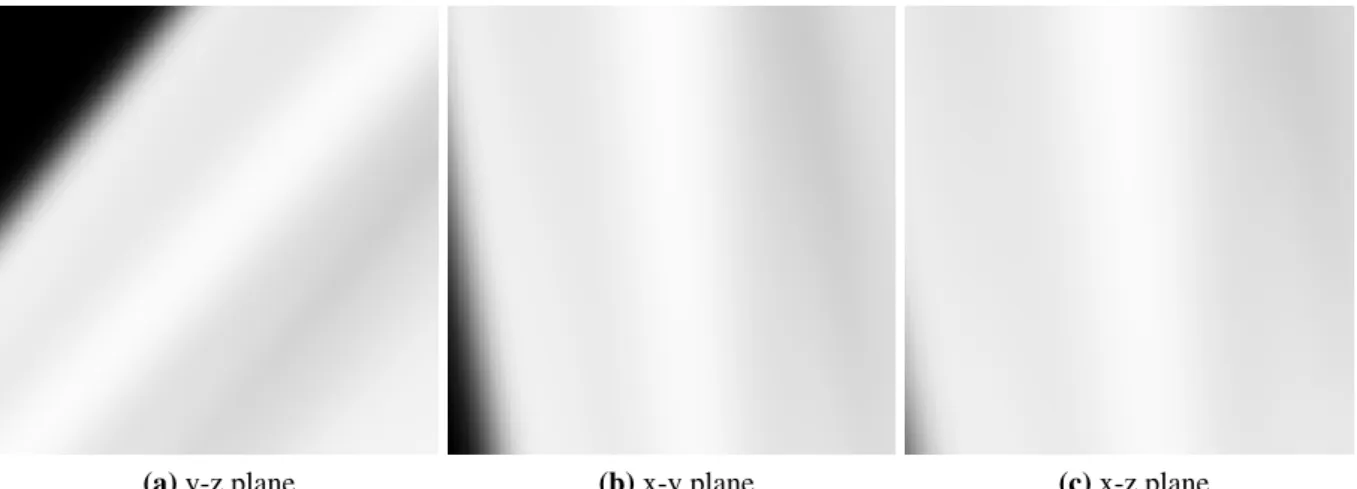Figure 6.4: The resulting transformation using a overtrained network using binary cross entropy with logits loss

Figur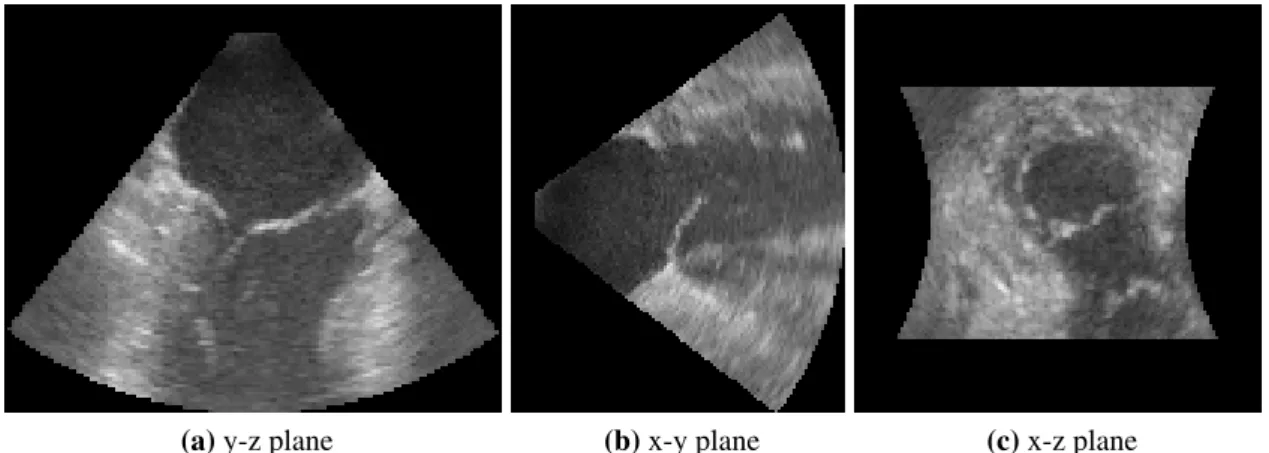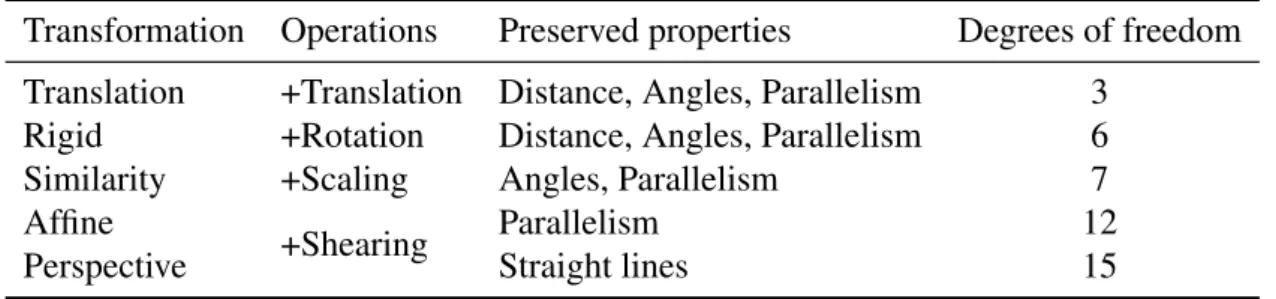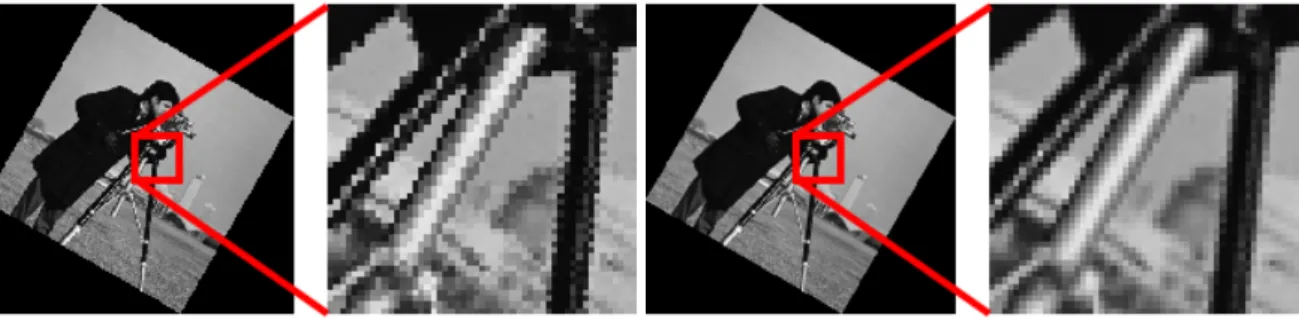+7

Referanser

RELATERTE DOKUMENTER

Figure 6.5 also shows the error rate of the KNN algorithm applied directly on the dataset of images without using the windowed scattering transform (4.2).. For each size of training

The ICM build model macro was used to construct the two models based on the obtained alignment between the two previous recommended templates and the target (default

Analysen viser at de relative forskjellene mellom barn av innvandrere og etniske norske barn i utdanningsnivå og inntekt blir mindre etter matching av barns alder og

From Column (1) of Table 5 we see that the availability of cross-border shopping is estimated to lead to a 25% ((exp(−.294) − 1) ∗ 100) reduction of grocery store sales for

As a result, a matrix was built (Table 1 ) and used to generate the PLS model by using the following different peptide descriptors: number of amino acids, number of negative

2.5D Multiscale Adaptive Histogram Equalization The algorithm described in [Jin04] for multiscale adaptive histogram equalization (MAHE) of CT images enhances low contrast features

More recently, also images acquired with different aperture masks but constant focus were used to solve the ambiguity between blur and texture [ZLN09], (CAP).. Thereby, careful

a) En av grunnelementene innen rational choice er antagelsen om metodologisk individualisme. Forklar dette begrepet, og beskriv hvordan man med en antagelse om

Hindmoor (2006) fremsetter i sin bok Rational Choice fire andre grunnantagelser som anvendes innen rational choice: bruk av modeller, antagelse om rasjonalitet,

Velg ditt språk

Nettstedet vil bli oversatt til språket du velger.

Foreslåtte språk for deg:

Annet: